## Example Questions

### Example Question #31 : Geometry

What is the perimeter of a pie piece if the pie is sliced into 40 degree pieces and its area is 361π?

40.1π

2.1

38π/9

38π

38 + 38π/9

38 + 38π/9

Explanation:

The perimeter of a given pie-piece will be equal to 2 radii plus the outer arc (which is a percentage of the circumference). For our piece, this arc will be 40/360 or 1/9 of the circumference. If the area is 361π, this means πr2 = 361 and that the radius is 19. The total circumference is therefore 2 * 19 * π or 38π.

The total perimeter of the pie piece is therefore 2 * r + (1 / 9) * c = 2 * 19 + (1/9) * 38π = 38 + 38π/9

### Example Question #32 : Geometry

What is the length of the arc of a circle with radius 10 that traces a 50 degree angle?

25π

25π/29

20π/9

25π/7

25π/9

25π/9

Explanation:

length of an arc = (degrees * 2πr)/360 = (50 * 2π * 10)/360 = 25π/9

### Example Question #33 : Geometry

An ant walks around the edge of circular pizzas left on the counter of a pizza shop. On most days, it is shaken off the pizza before it manages to walk the complete distance.

Quantity A: The distance covered by the ant when walking over four slices of a pizza with a diameter ofandequally-sized pieces.

Quantity B: The distance covered by the ant when walking over a complete personal pizza with a diameter ofinches.

What can we say about the two quantities?

The relationship between the two quantities cannot be determined.

Quantity B is larger.

Quantity A is larger.

The two quantities are equal.

Quantity B is larger.

Explanation:

Let's compute each quantity.

Quantity A

This is a little bit more difficult than quantity B.  It requires us to compute an arc length, for the ant does not walk around the whole pizza; however, this is not very hard. What we know is that the ant walks around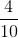, or, of the pizza.

Now, we know the circumference of a circle isor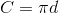For our example, let's use the latter. Since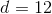, we know: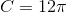However, our ant walks around only part of this, namely: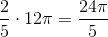Quantity B

This is really just a matter of computing the circumference of the circle.  For our value, we know this to be: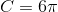Now, we can compare these by taking quantity A and reducing the fraction to be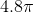. Thus, we know that Quantity B is larger than Quantity A.

Tired of practice problems?

Try live online GRE prep today.